# Bayesian Posterior Sampling

When using Bayesian posterior sampling, we want to the posterior, $${\rm P}(M(\vec{\theta}) \vert D)$$, of the model ($$M(\vec{\theta})$$) given one or more datasets ($$D$$) (i.e., plugin instances) and one model containing one or more sources with free parameters $:nbsphinx-math:vec{theta}$ given a likelihood $$L(\vec{\theta}) = {\rm P}(D \vert M(\vec{\theta}))$$ and prior $${\rm P}(\theta)$$ on the parameters. Only the simplest posteriors allow for an analytic solution, thus we must rely on Monte Carlo or nested sampling methods to sample the posterior.

In 3ML, we currently provide two popular posterior sampling methods: emcee and MULTINEST. If you installed via conda, both packages are available, otherwise, only emcee is included.

## emcee

emcee is an extensible, pure-Python implementation of Goodman & Weare’s Affine Invariant Markov chain Monte Carlo (MCMC) Ensemble sampler.” It uses multiple “walkers” to explore the parameter space of the posterior. For a complete understanding of the capabilites and limitations, we recommend a thorough reading of Goodman & Weare (2010). Nevertheless we emphasize these N points to keep in mind:

• emcee is for unimodal parameter estimation

• for complex likelihoods and marginal likelihood integration, check out thermodynamic integration (documentation coming soon)

Let’s take a look at its usage for a simple likelihood.

:

import warnings

warnings.simplefilter("ignore")
import numpy as np

np.seterr(all="ignore")

:

{'divide': 'warn', 'over': 'warn', 'under': 'ignore', 'invalid': 'warn'}

:

%%capture
from threeML import *
from threeML.bayesian.tutorial_material import *

:

from jupyterthemes import jtplot

%matplotlib inline
jtplot.style(context="talk", fscale=1, ticks=True, grid=False)
silence_warnings()
set_threeML_style()


Let’s get a BayesianAnalysis object like the one we would have in a normal 3ML analysis. We use a custom function, prepared for this tutorial, which gives a BayesianAnalysis object having a very simple model with one free parameter ($$\mu$$), and with a likelihood having a very simple shape:

:

# This returns a BayesianAnalysis object with a simple likelihood function,
# and the corresponding Model instance. These objects are what you will have
# in a typical 3ML analysis. The Model contains one point source, named "test",
# with a spectrum called "simple"
bayes, model = get_bayesian_analysis_object_simple_likelihood()
bayes.set_sampler("emcee")
# Let's look at the likelihood function, which in this illustrative example
# has a very simple shape
_ = plot_likelihood_function(bayes)

08:43:01 INFO      sampler set to emcee                                                    bayesian_analysis.py:197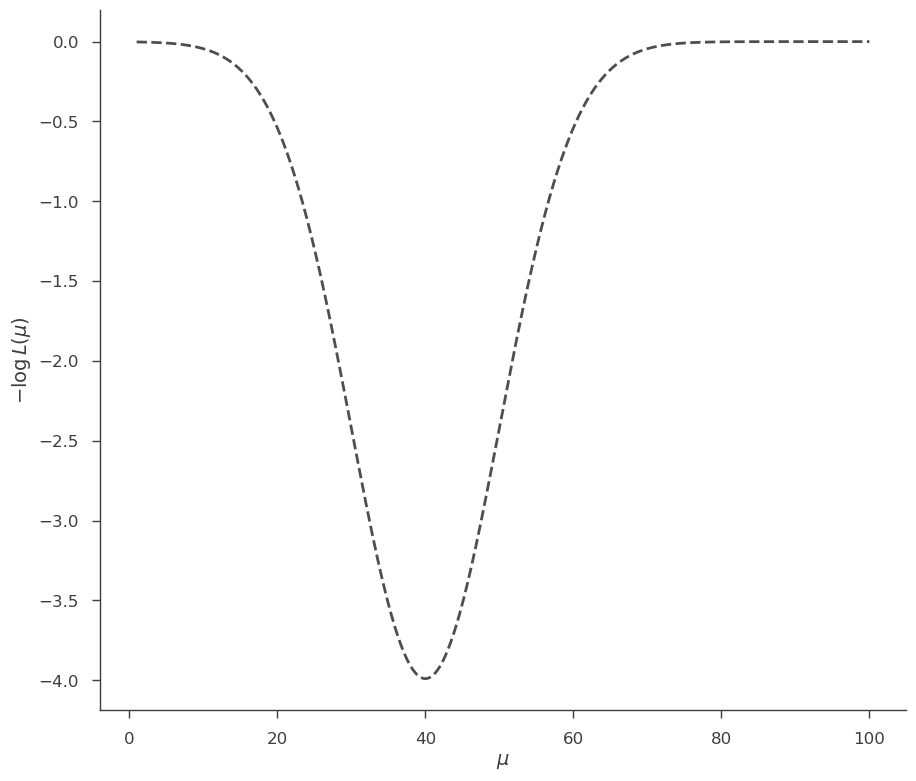We must define a prior fo $$\mu$$ in order to sample. Let’s use a uniform prior from 0 to 100. There are two ways to define this in 3ML:

:

# This directly assigns the prior
model.test.spectrum.main.shape.mu.prior = Uniform_prior(
lower_bound=1, upper_bound=100.0
)

# Alternatively, we can set an uniformative prior on the parameter's bounds
model.test.spectrum.main.shape.mu.set_uninformative_prior(Uniform_prior)


Ok, we are ready to sample. We will use only 5 walkers for this problem. We need to define a burn_in sample length which is how long we want to run the sampler to approach the target distribution so that we do not include these “learning” samples in our final results. Additionally, we need to declare the number of samples each walker will take.

:

n_walkers = 5
burn_in = 10
n_samples = 500

bayes.sampler.setup(n_iterations=n_samples, n_burn_in=burn_in, n_walkers=n_walkers)

res = bayes.sample()

08:43:03 INFO      Mean acceptance fraction: 0.7512000000000001                                emcee_sampler.py:157

         INFO      fit restored to maximum of posterior                                         sampler_base.py:178

         INFO      fit restored to maximum of posterior                                         sampler_base.py:178

Maximum a posteriori probability (MAP) point:


result unit
parameter
test.spectrum.main.Simple.mu (4.0 -0.8 +0.7) x 10 keV
Values of -log(posterior) at the minimum:


-log(posterior)
custom 3.989423
total 3.989423
Values of statistical measures:


statistical measures
AIC -9.978846
BIC -7.978846
DIC -3.926106
PDIC 2.024017
:

_ = plot_sample_path(bayes, truth=40.0, burn_in=n_walkers * burn_in)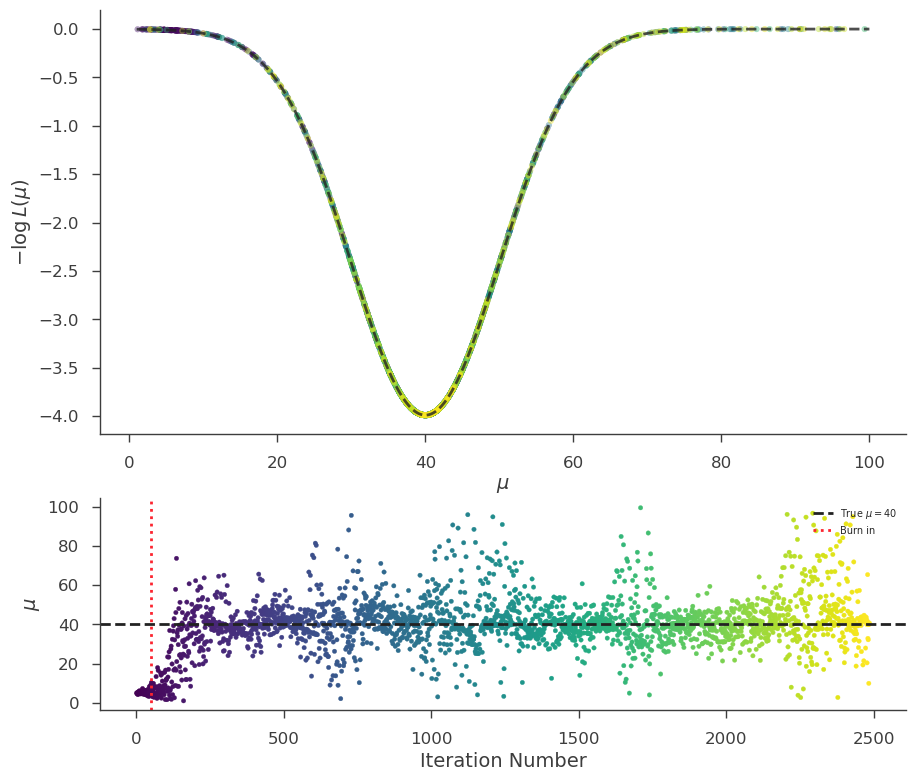We can see that we did not burn in the sampler long enough and we will have part of the burn in included in the results as can be seen in the marginal distribution of $$\mu$$:

:

_ = bayes.results.corner_plot()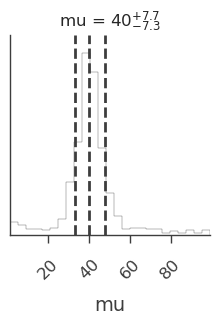We could easily run the sampler longer, but let’s reset the value of $$\mu$$ far from the target distribution and try more burn in samples.

:

model.test.spectrum.main.shape.mu = 99

n_walkers = 5
burn_in = 200
n_samples = 500

res = bayes.sample()

08:43:06 INFO      Mean acceptance fraction: 0.7407999999999999                                emcee_sampler.py:157

         INFO      fit restored to maximum of posterior                                         sampler_base.py:178

         INFO      fit restored to maximum of posterior                                         sampler_base.py:178

Maximum a posteriori probability (MAP) point:


result unit
parameter
test.spectrum.main.Simple.mu (4.0 -0.5 +1.0) x 10 keV
Values of -log(posterior) at the minimum:


-log(posterior)
custom 3.989423
total 3.989423
Values of statistical measures:


statistical measures
AIC -9.978846
BIC -7.978846
DIC -4.617780
PDIC 1.357529
:

_ = plot_sample_path(bayes, truth=40.0, burn_in=n_walkers * burn_in)
_ = bayes.results.corner_plot()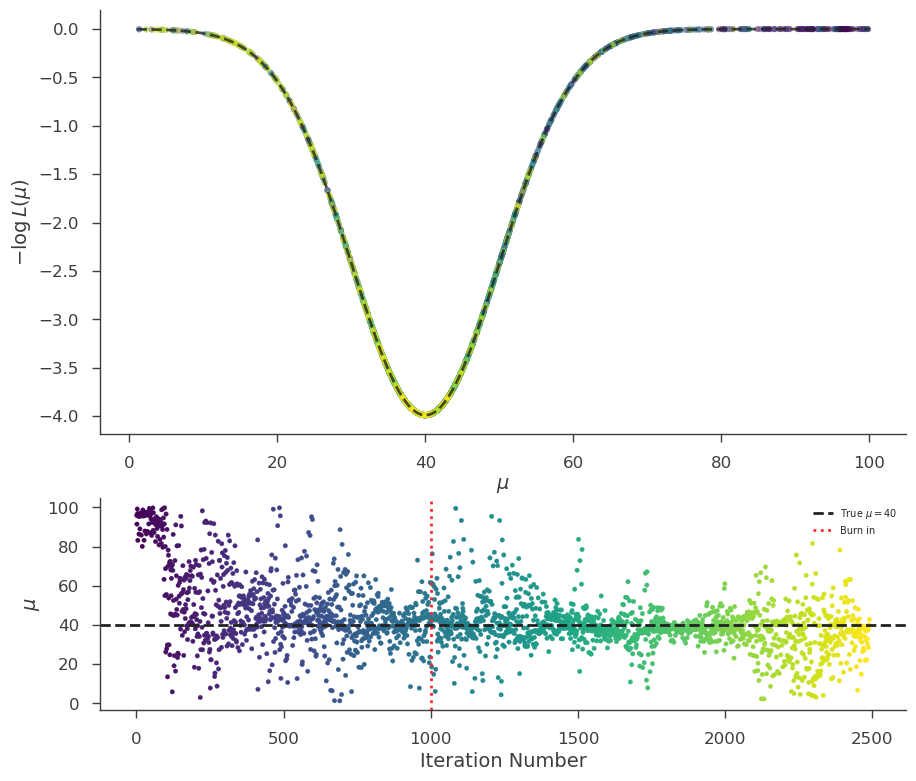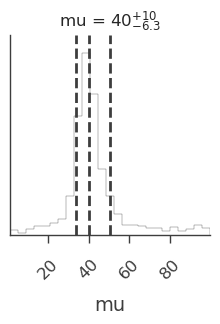The marginal distribution of the parameter $$\mu$$ is the answer for our parameter. It is the integral of the posterior over all other parameters, $$\vec{\phi}$$, given the data.

${\rm P}(\mu \vert D) = \int_{-\infty}^{\infty} {\rm d}\vec{\phi} \; {\rm P}( \mu, \vec{\phi}\vert D )$

Of course, in our simple model, there are no other parameters. The marginal natuarally includes the dependence of all other parameters and is only equivalent to MLE error estimates under assumptions of Gaussianity and linearity.

We can sometimes use point-descriptors for the marginal, e.g., mean, median, etc., but when the marginal distribution is not easily symmetric, it can be more descriptive to plot it or speak of its highest posterior density interval:

:

this_mu = bayes.results.get_variates("test.spectrum.main.Simple.mu")

print(this_mu.highest_posterior_density_interval(cl=0.68))
print(this_mu.highest_posterior_density_interval(cl=0.95))

(33.08653498305065, 47.40165343448834)
(24.79351931783488, 91.04317227323415)


### Priors

We have not paid much attention to our prior choice. So far, we have used an uninformative prior (except that we know in our example the range over which the parameter can be found), but what happens if we limit our prior such that we would never find the target distribution?

Let’s set a uniform prior such that $$\mu \in \{80-100 \}$$.

:

model.test.spectrum.main.shape.mu = 99
model.test.spectrum.main.shape.mu.prior = Uniform_prior(lower_bound=80, upper_bound=100)

n_walkers = 2
burn_in = 200
n_samples = 1000

bayes.sampler.setup(n_iterations=n_samples, n_burn_in=burn_in, n_walkers=n_walkers)
res = bayes.sample()

08:43:10 INFO      Mean acceptance fraction: 0.8245                                            emcee_sampler.py:157

         INFO      fit restored to maximum of posterior                                         sampler_base.py:178

         INFO      fit restored to maximum of posterior                                         sampler_base.py:178

Maximum a posteriori probability (MAP) point:


result unit
parameter
test.spectrum.main.Simple.mu (8.00 +0.28 +1.6) x 10 keV
Values of -log(posterior) at the minimum:


-log(posterior)
custom 0.001329
total 0.001329
Values of statistical measures:


statistical measures
AIC -2.002659
BIC -0.002659
DIC -0.000710
PDIC -0.000333
:

_ = plot_sample_path(bayes, truth=40.0, burn_in=n_walkers * burn_in)
_ = bayes.results.corner_plot()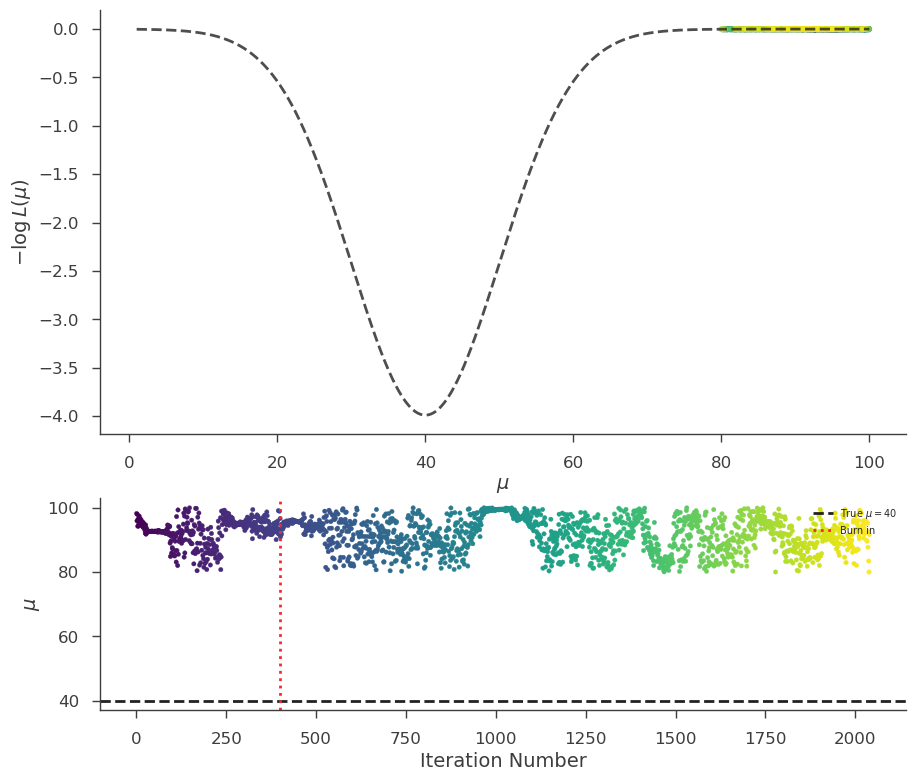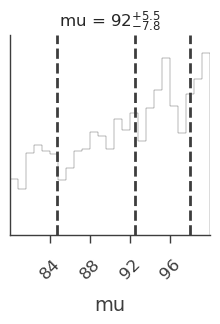We see that the over this range, the marginal distribution is the same as the prior, i.e., uniformative. The “data” have not updated our information about $$\mu$$ and thus we cannot conclude anything. This is by design in our example, but if we were to believe that $$\mu$$ had to be found in the interval $$\mu \in\{80-100\}$$, the data have not helped us to determine anything.

Let’s now look at setting a more informative prior on $$\mu$$. Suppose from either other measurements or physical insight, we are sure $$\mu\sim 30$$ with some uncertainty. Then we can impose a Gaussian prior over $$\mu$$.

:

model.test.spectrum.main.shape.mu = 99
model.test.spectrum.main.shape.mu.bounds = (None, None)
model.test.spectrum.main.shape.mu.prior = Gaussian(mu=25, sigma=5)

:

n_walkers = 2
burn_in = 200
n_samples = 1000

bayes.sampler.setup(n_iterations=n_samples, n_burn_in=burn_in, n_walkers=n_walkers)
res = bayes.sample()

08:43:14 INFO      Mean acceptance fraction: 0.8155                                            emcee_sampler.py:157

         INFO      fit restored to maximum of posterior                                         sampler_base.py:178

         INFO      fit restored to maximum of posterior                                         sampler_base.py:178

Maximum a posteriori probability (MAP) point:


result unit
parameter
test.spectrum.main.Simple.mu (3.1 -0.5 +0.4) x 10 keV
Values of -log(posterior) at the minimum:


-log(posterior)
custom -0.587521
total -0.587521
Values of statistical measures:


statistical measures
AIC -0.824957
BIC 1.175043
DIC 3.054058
PDIC 0.938684

Our prior information has biased the results to slightly lower values of $$\mu$$. Again, this is only to illustrate how to use different priors and their effects on the recovered results.

:

_ = plot_sample_path(bayes, truth=40.0, burn_in=n_walkers * burn_in)
_ = bayes.results.corner_plot()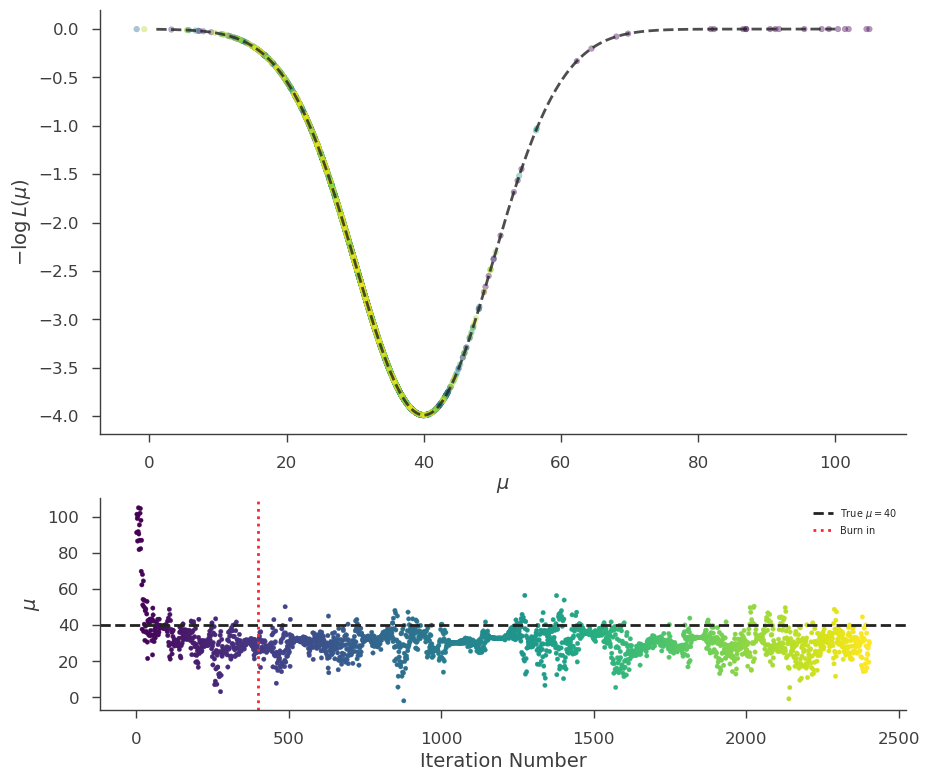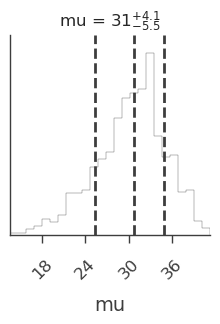## Complex Likelihood

We now examine a more complex likelihood.

:

bayes, model = get_bayesian_analysis_object_complex_likelihood()


## MULTINEST

MULTINEST is a nested sampling algorithm that is designed to integrate the the posterior to obtain the marginal likelihood. For details on the algorithm see Feroz et al. (2009), Feroz et al. (2013), and for details on the input arguments for the python interface we implement, see the documentation of pymultinest. If you find these algorithms useful for your research, please cite the originals authors!

Let’s sample the complex likelihood from above with MULTINEST using

:

bayes.set_sampler("multinest")

model.test.spectrum.main.shape.mu.prior = Uniform_prior(lower_bound=1, upper_bound=100)

_ = plot_likelihood_function(bayes)

bayes.sampler.setup(n_live_points=400, resume=False)

res = bayes.sample()

08:43:16 INFO      sampler set to multinest                                                bayesian_analysis.py:197

 *****************************************************
MultiNest v3.10
Copyright Farhan Feroz & Mike Hobson
Release Jul 2015

no. of live points =  400
dimensionality =    1
*****************************************************
ln(ev)=   10.011774357067818      +/-   8.7377066342742712E-002
Total Likelihood Evaluations:         2627
analysing data from chains/fit-.txt
Sampling finished. Exiting MultiNest

08:43:19 INFO      fit restored to maximum of posterior                                         sampler_base.py:178

         INFO      fit restored to maximum of posterior                                         sampler_base.py:178

Maximum a posteriori probability (MAP) point:


result unit
parameter
test.spectrum.main.Complex.mu (5.999 -2.5 +0.007) x 10 keV
Values of -log(posterior) at the minimum:


-log(posterior)
custom 13.83816
total 13.83816
Values of statistical measures:


statistical measures
AIC -29.676319
BIC -27.676319
DIC -44.895206
PDIC -18.747280
log(Z) 4.348058:

_ = plot_sample_path(bayes)
_ = bayes.results.corner_plot()

No artists with labels found to put in legend.  Note that artists whose label start with an underscore are ignored when legend() is called with no argument.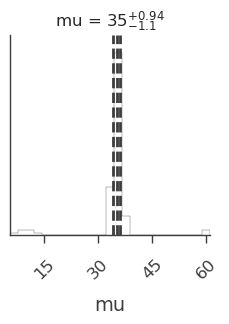MULTINEST has fully sampled the likelihood and broken apart the modes. Cleary point-descriptors of the results will be inefficent, but we use the output files of MULTINEST to do multi-modal analysis. For details, consult the MULTINEST documentation.

## UltraNest

Finally we can try with ultranest which is another nested sampling algorithm.

:

bayes.set_sampler("ultranest")
bayes.sampler.setup()

res = bayes.sample(quiet=True)

08:43:22 INFO      sampler set to ultranest                                                bayesian_analysis.py:197

[ultranest] Sampling 400 live points from prior ...

[ultranest] Explored until L=1e+01
[ultranest] Likelihood function evaluations: 3885
[ultranest]   logZ = 9.809 +- 0.06475
[ultranest] Effective samples strategy satisfied (ESS = 1389.9, need >400)
[ultranest] Posterior uncertainty strategy is satisfied (KL: 0.46+-0.08 nat, need <0.50 nat)
[ultranest] Evidency uncertainty strategy is satisfied (dlogz=0.07, need <0.5)
[ultranest]   logZ error budget: single: 0.09 bs:0.06 tail:0.02 total:0.07 required:<0.50
[ultranest] done iterating.

08:43:30 INFO      fit restored to maximum of posterior                                         sampler_base.py:178

         INFO      fit restored to maximum of posterior                                         sampler_base.py:178

:

_ = plot_sample_path(bayes)
_ = bayes.results.corner_plot()

No artists with labels found to put in legend.  Note that artists whose label start with an underscore are ignored when legend() is called with no argument.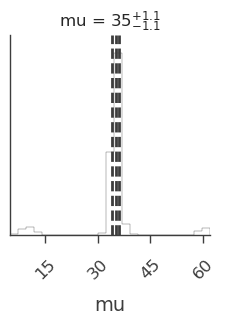## Accessing samples and error propagation

Error propagation with posterior samples is straight forward. Say we have a function $$f(\mu) = Sin(\mu)$$. For the complex likelihood, we would be out of luck if we were using MLE. However, we can directly calculate $$f(\mu)$$ with the samples from the posterior.

:

def f(mu):
return mu**2

this_mu = bayes.results.get_variates("test.spectrum.main.Complex.mu")

f_mu = f(this_mu)

print(f_mu)

fig, ax = plt.subplots()

ax.hist(f_mu.samples, bins=100)

_ = ax.set_xlabel(r"f($\mu$)")

equal-tail: (1.28 -0.08 +2.3) x 10^3, hpd: (1.28 -0.07 +2.3) x 10^3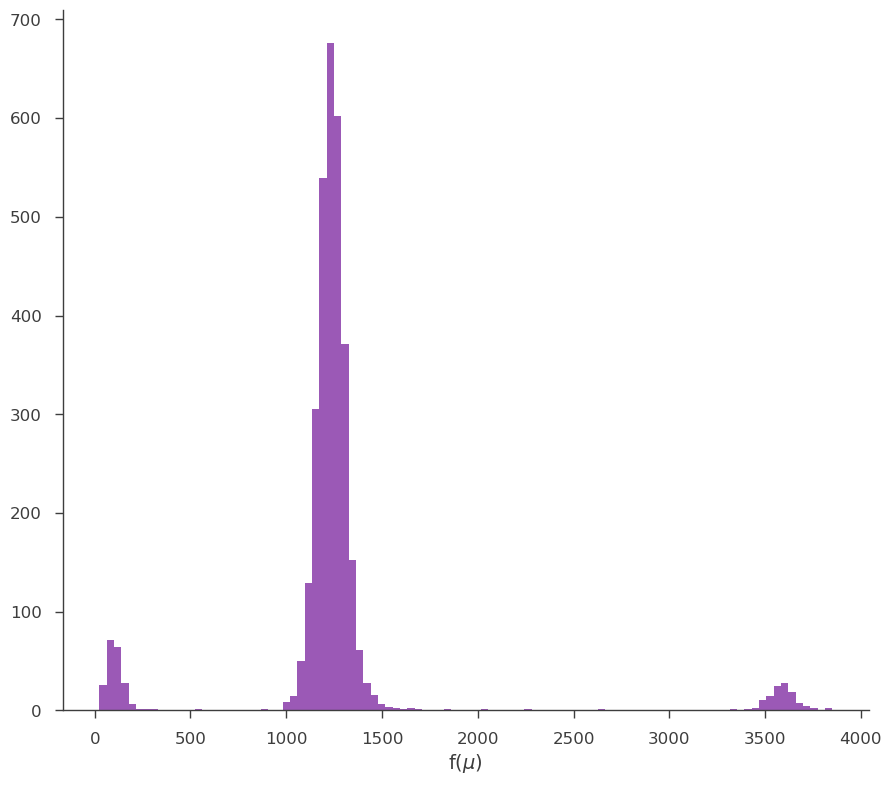As with any approach, the Bayesian capabilities of 3ML are a tool. Understanding and proper use of the posterior and marginal distributions requires special care. For further reading on the Bayesian analysis and its applications to spectral analysis check out the following resources:

[ ]: